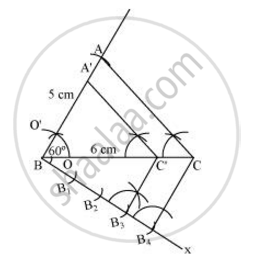# Construct a triangle ABC in which BC = 6 cm, AB = 5 cm and ∠ABC = 60°. Then construct another triangle whose sides are3/4 times the corresponding sides of ΔABC. - Mathematics

Construct a triangle ABC in which BC = 6 cm, AB = 5 cm and ∠ABC = 60°. Then construct another triangle whose sides are3/4 times the corresponding sides of ΔABC.

#### Solution

Following steps are involved in the construction of a ΔA'BC' whose sides are3/4 of the corresponding sides of ΔABC:

Step 1

Draw a ΔABC with sides BC = 6 cm and AB = 5 cm and ∠ABC = 60°.

Step 2

Draw a ray BX making an acute angle with BC on the opposite side of vertex A.

Step 3

Locate 4 points (as 4 is greater in 3 and 4) B1, B2, B3 and B4 on line segment BX.

Step 4

Join B4C and draw a line through B3 parallel to B4C intersecting BC at C'.

Step 5

Draw a line through C' parallel to AC intersecting AB at A'. ΔA'BC' is the required triangle.Justification

The construction can be justified by proving

A'B=3/4AB, BC'=3/4BC, A'C'=3/4AC

In ΔA'BC' and ΔABC,

∠A'C'B = ∠ACB (Corresponding angles)

∠A'BC' = ∠ABC (Common)

∴ ΔA'BC' ∼ ΔABC (AA similarity criterion)

=>(A'B)/(AB)=(BC')/(BC)=(A'C')/(AC)

In ΔBB3C' and ΔBB4C,

∠B3BC' = ∠B4BC  (Common)

∠BB3C' = ∠BB4C (Corresponding angles)

∴ ΔBB3C' ∼ ΔBB4C (AA similarity criterion)

=>(BC')/(BC)=(BB_3)/(BB_4)

=>(BC')/(BC)=3/4

From (1) and (2), we obtain

(A'B)/(AB)=(BC')/(BC)=(A'C')/(AC)=3/4

=>A'B=3/4AB, BC'=3/4BC, A'C'=3/4AC

This justifies the construction.

Concept: Division of a Line Segment
Is there an error in this question or solution?
2015-2016 (March) Delhi Set 2

Share